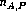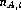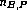Next: Computation Up: Testing Team Performance Previous: The Problem: Combining

# The Permutation Test

The permutation test is based on a pair of documents, say A and B. Let us assume we have=++PBR subjects andNASA subjects for document A. Similarly, assume we havePBR subjects andNASA subjects for document B. Finally, we require=and=. This just means that each subject used a different technique on the two documents.

Now we can form all possible combinations of teams for each of the four combinations of techniques and documents, much as explained in the first approach mentioned above. For each of these four resulting sets of teams, we compute the mean defect detection rates:,,and. Then, a test statistic to indicate the relative advantage of PBR can be defined as

(-) + (-)
However, as explained above, we cannot do much more here because the data points are not independent - an assumption made by most relevant tests of significance.

Let us assume that there is a difference between the two techniques we are comparing. Now, for one document, imagine we can take a PBR reader and just pretend he used the NASA technique, and at the same time pick a NASA reader and pretend he used PBR. For the other document we do a similar ``swap'' involving the same two people. Then we compute the test statistic mentioned above. If our assumption about PBR holds, this ``dillution'' of the groups of people will result in a different value of the test statistic. On the other hand, if there is no difference between the techniques, the resulting differences in the test statistic will be random in either direction.

Pretending that a PBR subject uses the usual technique is straightforward. But the opposite case requires some additional decisions to be made. The problem is that there are three possible roles for a PBR reader to use. Our solution to this was to introduce a fourth dummy role which is only assigned to these subjects. Then, a PBR team can consist of any combination of three subjects from a group of one designer, one tester, one user, and any number of dummy-role readers. Thus, an extesively dilluted PBR team can consist of e.g. three dummy-role readers.

The actual result is found by ranking the test statistic to form a frequency distribution, and then finding the percentile of the data point which represents the actual (non-dilluted) reults. This percentile directly represents the significance of the test.Next: Computation Up: Testing Team Performance Previous: The Problem: Combining

Generated by latex2html-95.1
Mon Jun 24 13:58:35 EDT 1996

Web Accessibility